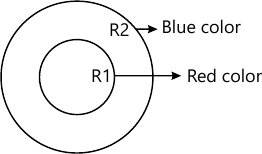MORE IN Computer Graphics & Virtual Reality
MU Information Technology (Semester 5)
Computer Graphics & Virtual Reality
December 2014
Total marks: --
Total time: --
INSTRUCTIONS
(1) Assume appropriate data and state your reasons
(2) Marks are given to the right of every question
(3) Draw neat diagrams wherever necessary

1 (a) If 8 bits per pixel, per color scheme is used for a RGB display device, How much memory is required to hold picture data worth one screen, if the resolution is 800×600. If the refresh rate is 50Hz, How much memory is required to bold picture data for duration of one sec.
5 M
1 (b) Prove that he two successive rotation Transformations are additive.
5 M
1 (c) Explain different ways of performing text clipping.
5 M
1 (d) What is VRML, what is the meaning of six degrees of freedom in 3D graphics. How to define any shape (say cylinder) in VRML.
5 M

2 (a) Given 4 control points (10,10), (15,15), (20,15) and (30,10), find the points to plot bezier curve by using step size as 0.2.
10 M
2 (b) Explain Boundary fill and flood fill Algorithm. Which algorithm cannot be used to fill the Region R2 which is bounded by Blue color and Red color boundary, Justify.10 M

3 (a) Find sequence of Transforming required to rotate a solid object w.r.t. a line y=mx+c, in anticlockwise manner by angle ?.
10 M
3 (b) Explain Morphing and warping mechanism.
10 M

4 (a) What are the important components of VR system. Explain different types of VR systems.
10 M
4 (b) What is projection what are its diferent types? Derive transformation Matrix for oblique projection.
10 M

5 (a) Explain Geometric and kinematic modelling in detail.
10 M
5 (b) Explain an algorithm which uses parametric equation for the propose of line clipping using the same algorithm, find the coordinates of the line segment A(10,10), B(70, 40), after it is clipped against a window with two diagonal vertices at (20,20) (40, 50).
10 M

Write short notes on: (any two)
6 (a) Fractals
10 M
6 (b) Applications of VR
10 M
6 (c) 3D position Trackers.
10 M

More question papers from Computer Graphics & Virtual Reality